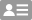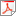# SILVIA FALLETTAAssociate Professor

+39 0110907506 / 7506 (DISMA)

Master degree in Mathematics, University of Genova, 1997.

INdAM (Istituto Nazionale di Alta Matematica) grant for one year study at Dip. di Matematica in Milano, 1998.

PhD in Mathematics and Scientific Calculus, University of Pavia, 2003.

Temporary research scientist at University of Pavia, January 2003-December 2003.

Temporary research scientist at University of Milano Bicocca, January 2004-December 2005.

Post-Doc position at I.A.N.S Stuttgart University, Germany, January-March 2006.

Temporary research scientist at University of Milano, June 2006-June 2007.

Permanent researcher at Dip. Matematica of Politecnico of Torino, October 2007 - March 2021.

Associate Professor at Dip. Matematica of Politecnico of Torino, March 2021-now.

Research interests:

Construction of Wavelet basis on the interval.

Non conforming domain decomposition methods for PDEs:

* Mortar techniques.

* Coupling of Wavelets and Finite Elements.

* The Three Fields formulation

* Nested domains.

Scientific branch MAT/08 - ANALISI NUMERICA(Area 0001 - Scienze matematiche e informatiche)
Curriculum Curriculum file(310 KB)
Identifiers ORCID: 0000-0002-4957-3972 Scopus Author ID: 6504796979
Research topics Numerical solution of acoustic and elastic wave propagation problems, both in frequency and time domain, defined in bounded and unbounded domains and described by partial differential equations (PDEs). Problems, whose formulation can be reduced to boundary integral equations, are considered. Among the classical examples, it is worth mentioning: i) the propagation and the simple/multiple scattering of acoustic waves in non-viscous fluids, in presence of obstacles of arbitrary shape; ii) the propagation of elastic (seismic) waves in homogeneous or multi-layered media and that of electromagnetic waves. The presence of sources, even located far away from the spatial domain of interest, of non-trivial initial data, and of Dirichlet, Neumann or mixed boundary conditions are considered. By using a proper boundary integral formulation, it is possible to determine directly the solution of the problem at any desired point. The same integral formulation represents a non-reflecting boundary condition that can be defined on an artificial boundary of arbitrary shape, thus allowing to solve the problem on a bounded spatial domain of interest. The solution of the reformulated problem is obtained by coupling a boundary element discretization (BEM) of the above mentioned integral equation with a classical finite element method (FEM) or a finite difference method, or by means of the virtual element method (VEM). The applied numerical techniques entail: • collocation and Galerkin methods for the numerical solution of integral formulation of PDEs and efficient quadrature formulas for the computation of weakly and strongly singular integrals therein involved. This last issue is particularly important for the efficacy and the accuracy of the numerical method applied to the integral equation; • convolution quadrature formulas for space-time boundary element methods; • wavelet techniques for the sparsification of the matrices associated with linear systems arising from the space-time discretization of the integral operators involved in the integral reformulation of PDEs.
Skills and keywords

### ERC sectors

PE1_20 - Application of mathematics in sciences PE1_17 - Numerical analysis

### Keywords

Acoustic waves Advanced numerical methods Convolution integrals Elasticity Integral equation method Integral equations Seismology